Find solution of equations- Equations given

Chapter 4 Class 12 Determinants
Concept wise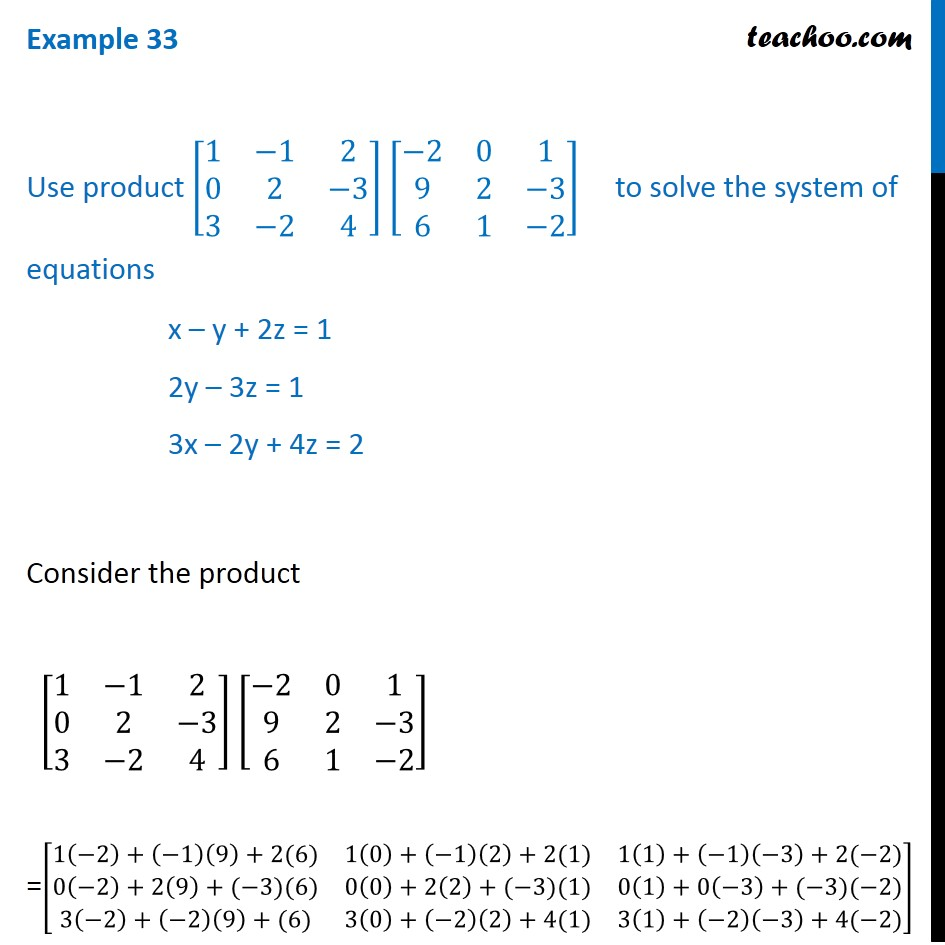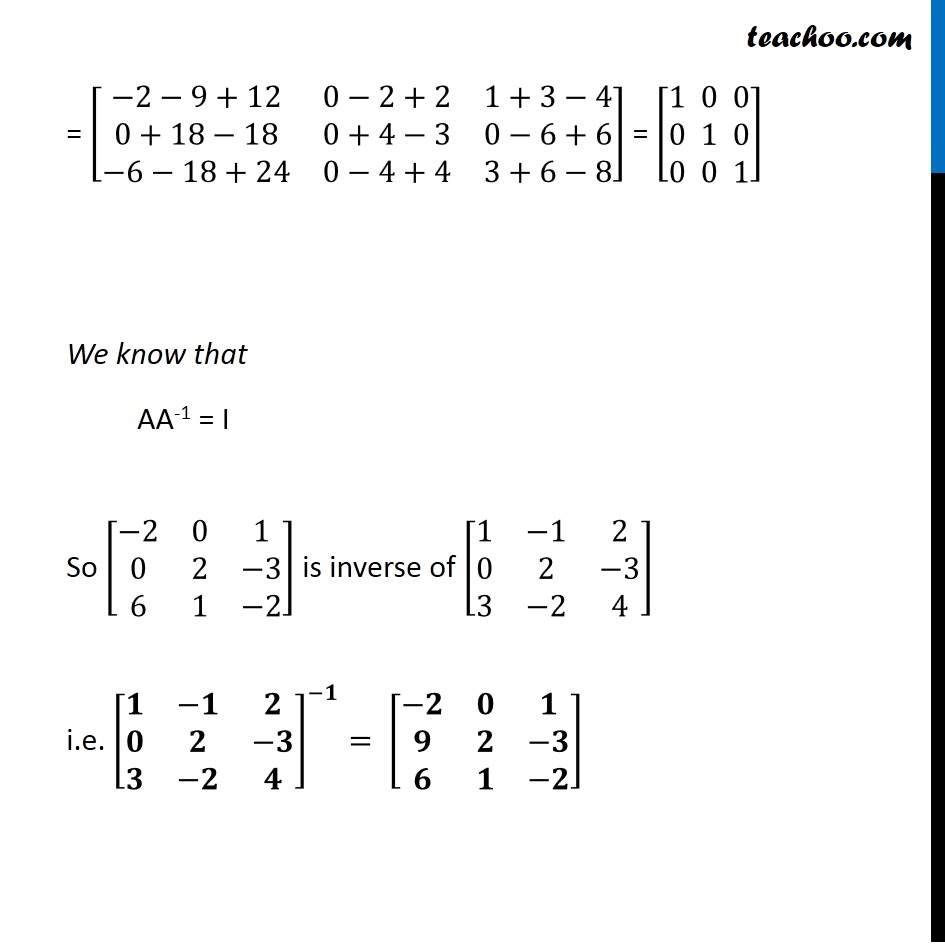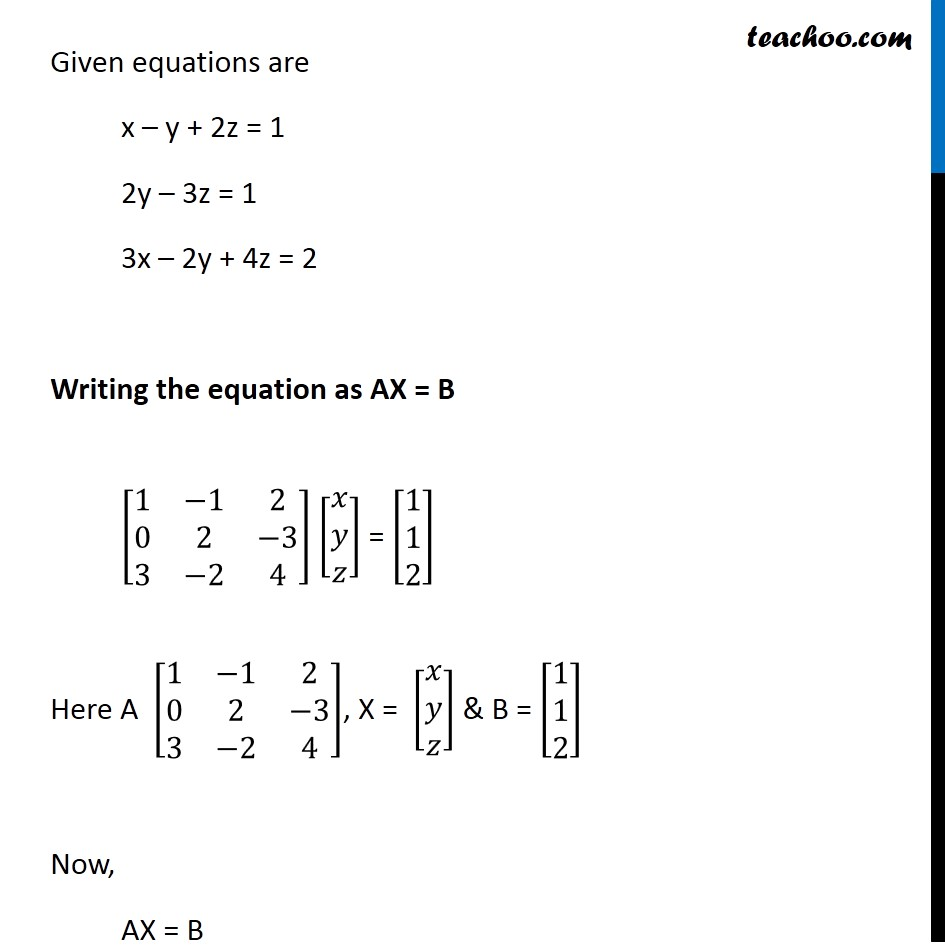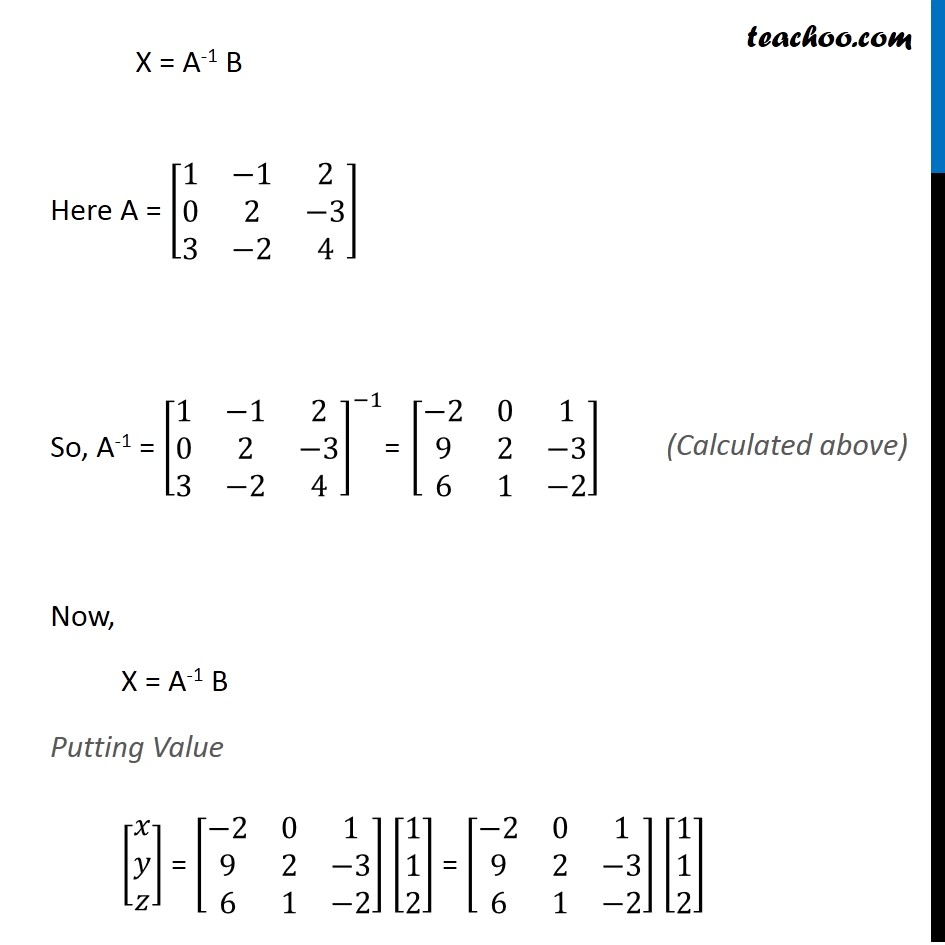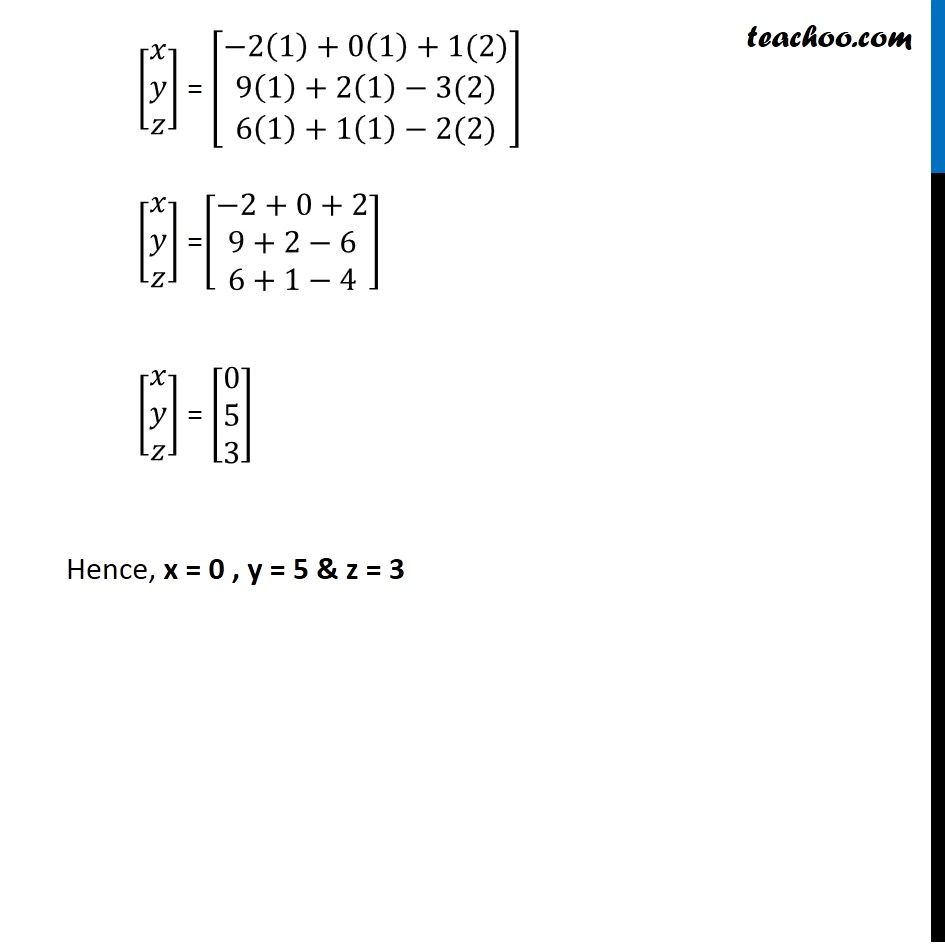Learn in your speed, with individual attention - Teachoo Maths 1-on-1 Class

### Transcript

Example 19 Use product [■8(1&−1&[email protected]&2&−[email protected]&−2&4)] [■8(−2&0&[email protected]&2&−[email protected]&1&−2)] to solve the system of equations x – y + 2z = 1 2y – 3z = 1 3x – 2y + 4z = 2 Consider the product [■8(1&−1&[email protected]&2&−[email protected]&−2&4)] [■8(−2&0&[email protected]&2&−[email protected]&1&−2)] =[■8(1(⤶7−2)+(⤶7−1)(9)+2(6)&1(0)+(−1)(2)+2(1)&1(1)+(−1)(−3)+2(−2)@0(−2)+2(9)+(−3)(6)&0(0)+2(2)+(−3)(1)&0(1)+0(−3)+(−3)(−2)@3(−2)+(−2)(9)+(6)&3(0)+(−2)(2)+4(1)&3(1)+(−2)(−3)+4(−2))] = [■8(−2−9+12&0−2+2&1+3−[email protected]+18−18&0+4−3&0−6+6@−6−18+24&0−4+4&3+6−8)] = [■8([email protected]@0)" " ■8([email protected]@0)" " ■8([email protected]@1)] We know that AA-1 = I So [■8(−2&0&[email protected]&2&−[email protected]&1&−2)] is inverse of [■8(1&−1&[email protected]&2&−[email protected]&−2&4)] i.e. [■8(𝟏&−𝟏&𝟐@𝟎&𝟐&−𝟑@𝟑&−𝟐&𝟒)]^(−𝟏)= [■8(−𝟐&𝟎&𝟏@𝟗&𝟐&−𝟑@𝟔&𝟏&−𝟐)] Given equations are x – y + 2z = 1 2y – 3z = 1 3x – 2y + 4z = 2 Writing the equation as AX = B [■8(1&−1&[email protected]&2&−[email protected]&−2&4)] [■8(𝑥@𝑦@𝑧)] = [■8([email protected]@2)] Here A [■8(1&−1&[email protected]&2&−[email protected]&−2&4)], X = [■8(𝑥@𝑦@𝑧)] & B = [■8([email protected]@2)] Now, AX = B X = A-1 B Here A = [■8(1&−1&[email protected]&2&−[email protected]&−2&4)] So, A-1 = [■8(1&−1&[email protected]&2&−[email protected]&−2&4)]^(−1)= [■8(−2&0&[email protected]&2&−[email protected]&1&−2)] Now, X = A-1 B Putting Value [■8(𝑥@𝑦@𝑧)] = [■8(−2&0&[email protected]&2&−[email protected]&1&−2)] [■8([email protected]@2)] = [■8(−2&0&[email protected]&2&−[email protected]&1&−2)] [■8([email protected]@2)] (Calculated above) [■8(𝑥@𝑦@𝑧)] = [■8(−2(1)+0(1)+1(2)@9(1)+2(1)−3(2)@6(1)+1(1)−2(2))] [■8(𝑥@𝑦@𝑧)] =[■8(−[email protected]+2−[email protected]+1−4)] [■8(𝑥@𝑦@𝑧)] = [■8([email protected]@3)] Hence, x = 0 , y = 5 & z = 3